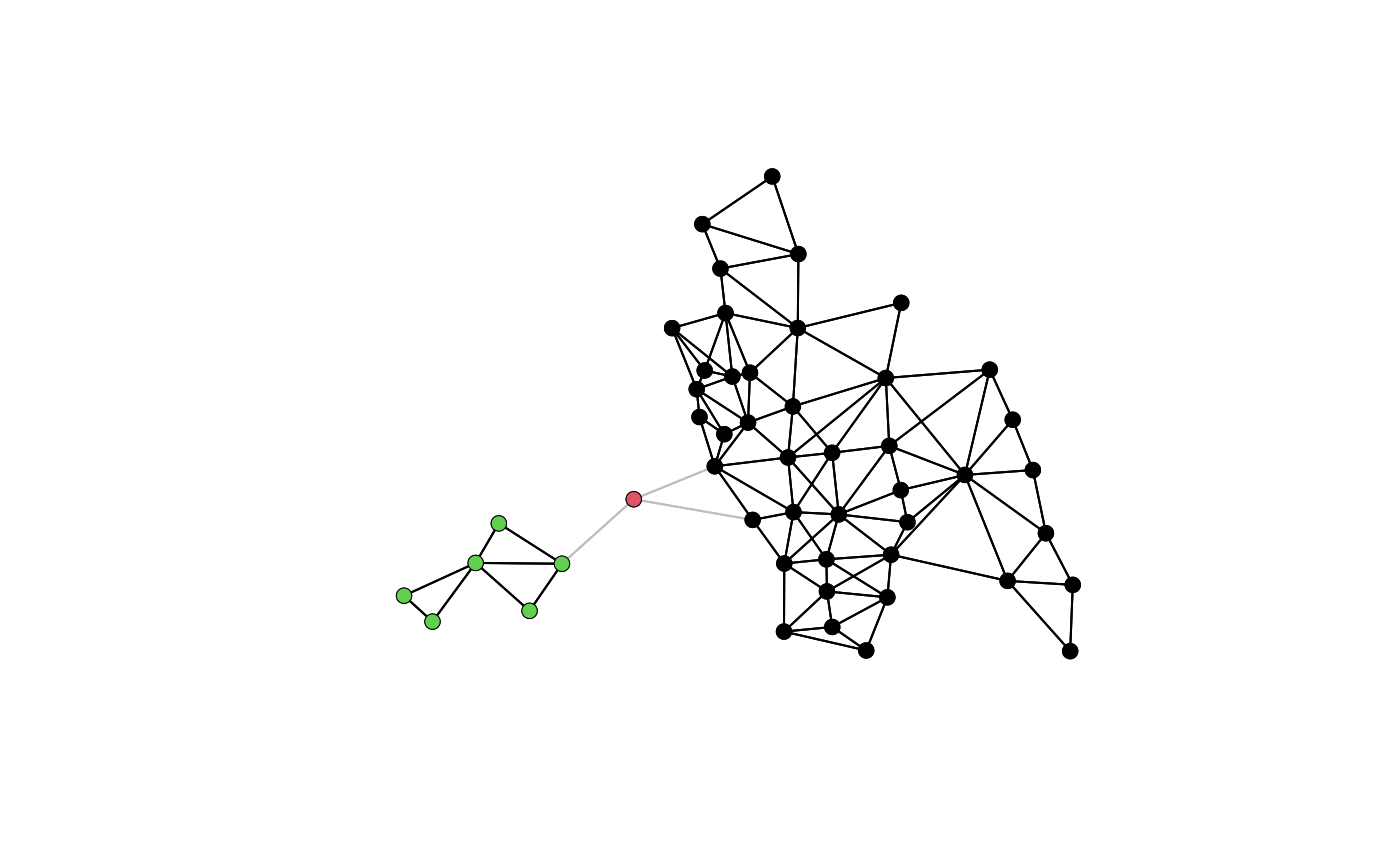n.comp.nb() finds the number of disjoint connected subgraphs in the graph depicted by nb.obj - a spatial neighbours list object.

n.comp.nb(nb.obj)

## Arguments

nb.obj

a neighbours list object of class nb

## Value

A list of:

nc

number of disjoint connected subgraphs

comp.id

vector with the indices of the disjoint connected subgraphs that the nodes in nb.obj belong to

## Author

Nicholas Lewin-Koh nikko@hailmail.net

plot.nb

## Examples

columbus <- st_read(system.file("shapes/columbus.shp", package="spData"), quiet=TRUE)
coords <- st_coordinates(st_centroid(st_geometry(columbus)))
plot(col.gal.nb, coords, col="grey")
res <- n.comp.nb(col2)
table(res$comp.id) #> #> 1 2 3 #> 42 1 6 plot(col2, coords, add=TRUE) points(coords, col=res$comp.id, pch=16)run <- FALSE
if (require(igraph, quietly=TRUE) && require(spatialreg, quietly=TRUE)) run <- TRUE
#>
#> Attaching package: ‘igraph’
#> The following objects are masked from ‘package:stats’:
#>
#>     decompose, spectrum
#> The following object is masked from ‘package:base’:
#>
#>     union
#>
#> Attaching package: ‘spatialreg’
#> The following objects are masked from ‘package:spdep’:
#>
#>     get.ClusterOption, get.VerboseOption, get.ZeroPolicyOption,
#>     get.coresOption, get.mcOption, set.ClusterOption,
#>     set.VerboseOption, set.ZeroPolicyOption, set.coresOption,
#>     set.mcOption
if (run) {
B <- as(nb2listw(col2, style="B", zero.policy=TRUE), "CsparseMatrix")
c1 <- clusters(g1)
print(c1$no == res$nc)
}
#>  TRUE
if (run) {
print(all.equal(c1$membership, res$comp.id))
}
#>  "names for target but not for current"
if (run) {
print(all.equal(c1$csize, c(table(res$comp.id)), check.attributes=FALSE))
}
#>  TRUE
if (run) {
W <- as(nb2listw(col2, style="W", zero.policy=TRUE), "CsparseMatrix")
print(all.equal(c1W$membership, res$comp.id, check.attributes=FALSE))